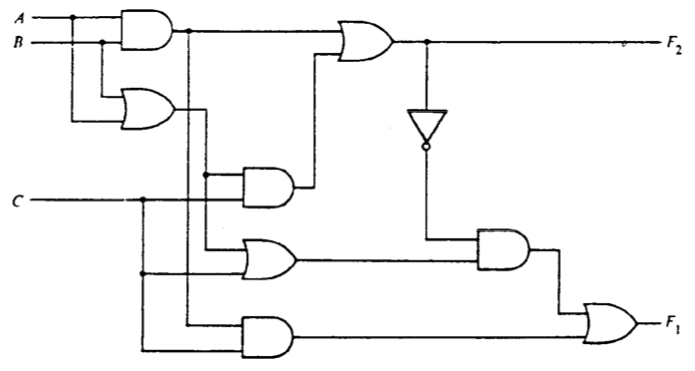pencil Work with digital logic gates.

# Exercises

Problems marked [Independent] must be completed without assistance from tutors or instructors.

1. [3 points] Write truth tables and unsimplified Boolean expressions for each of the output signals $F_1$ and $F_2$ in the following circuit. Create the boolean expressions via a direct translation of the circuit.2. [6 points] Draw unsimplified circuits to implement the following Boolean expressions. We use the apostrophe$’$ notation as an alternative to the overbar to indicate logical negation of the preceding term. The apostrophe binds tightly. For example, $AB’$ means $(A)(B’)$.

1. $(A + B)(A + B’)$
2. $ABC + A’B + ABC’$
3. [Independent] $A’B’ + A’BC’ + (A + C’)’$
3. [5 points] Write a Boolean expression and draw a circuit in sum-of-products form for the output $Y$ of this truth table.

 $A$ $B$ $C$ $Y$ 0 0 0 1 0 0 1 0 0 1 0 1 0 1 1 0 1 0 0 1 1 0 1 1 1 1 0 0 1 1 1 1
4. [15 points] Using elementary rules of Boolean algebra, find the simplest equivalent of the Boolean expressions below using only products, sums, and negation (i.e., no XOR). Show your derivation step by step. Label each step with the name of the rule you apply. You may find this sheet of Boolean laws useful.

1. $(A + B)(A + B’)$
2. $ABC + A’B + ABC’$
3. [Independent] $A’B’ + A’BC’ + (A + C’)’$
4. [Independent. This one is harder and serves as a teaser to motivate a useful technique we will learn later. Give it a try, but do not be concerned if you think you are stuck. ] $A’B’C’D’ + AB’C’ + AB’CD’ + ABD + A’B’CD’ + BC’D + A’$
5. [6 points] Draw two circuits that implement two-input XOR:

1. Using only 2-input NAND gates.
2. [Independent] Using only 2-input NOR gates.

In both circuits, use the smallest number of gates you can.